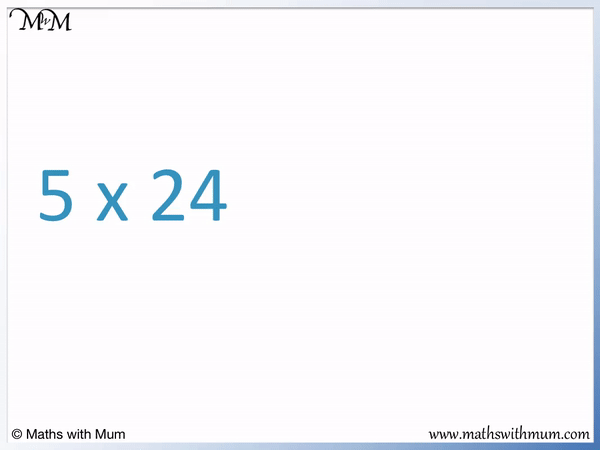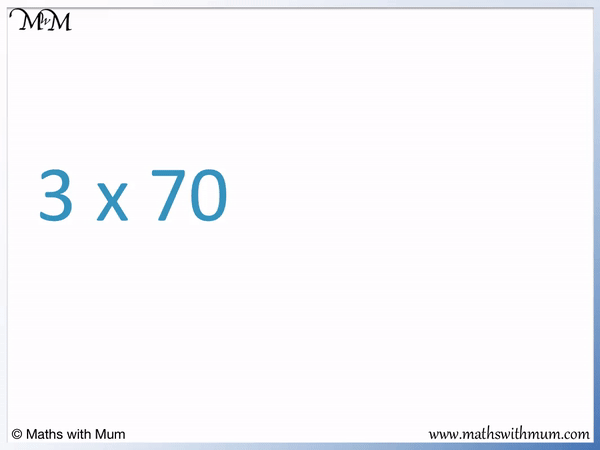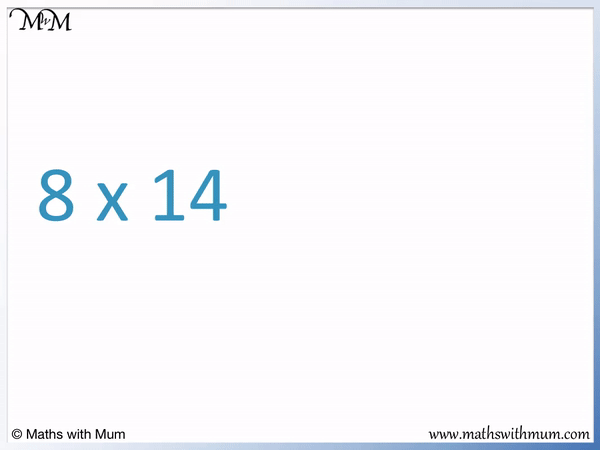# Multiplication Using Factors

Multiplication Using Factors• Factors are numbers that divide exactly into other numbers.
• Larger numbers can be written as their factors multiplied together.
• For example, 15 = 3 × 5. 3 and 5 are factors of 15.
• Instead of multiplying by 15, we can multiply by 3 and then by 5.
• 3 × 15 is the same as 3 × 3 × 5.
• 3 × 3 = 9 and then 9 × 5 = 45.
• Therefore, 3 × 15 = 45.
• This method is used to multiply by larger numbers.
• Multiplying by smaller numbers in stages is easier than multiplying by larger numbers in one go.
To multiply by a larger number, multiply by its factors.• 24 can be written as the product of its two factors, 4 × 6.
• 5 × 24 can be written as 5 × 4 × 6.
• 5 × 4 = 20 and 20 × 6 = 120.
• Therefore, 5 × 24 = 120.# Multiplication Using Factors

## How to Multiply Numbers Using Factors

A factor is a number that divides exactly into another number. Instead of multiplying by a larger number in one go, it can be easier to multiply by the factors that multiply to make this number. For example, to multiply 3 × 70, it can be easier to multiply 3 × 7 and then multiply by 10.

Choose two factors of the larger number which will be easiest to multiply by. For example, multiplying 3 × 7 × 10 is easier than multiplying 3 × 2 × 35. Even though 2 × 35 = 70, 35 is not an easy number to multiply by.

3 × 7 = 21 and this is a known fact from our times tables. It is then simple to multiply by 10 by placing a 0 digit on the end of 21.3 × 7 × 10 = 210 and so, 3 × 70 = 210.

Here is 5 × 6.

We can write 6 as 2 × 3.5 × 6 can be written as 5 × 2 × 3.

5 × 2 = 10 and then 10 × 3 = 30.

5 × 2 × 3 = 30 and so, 5 × 6 = 30.

Factors can be used to help us work out any multiplication. This method can also be used for teaching and learning the times tables. If you knew the 2 and 3 times tables but were still learning the 6 times tables, you can multiply 5 by 2 and then by 3 to work out 5 × 6.

We choose to do 5 × 2 × 3 rather than 5 × 3 × 2 because 5 × 2 = 10 and this is an easy number to multiply by. When teaching multiplication by using factors, try to find groups of easier multiplications.

Here is 7 × 9.

9 = 3 × 3 and so, multiplying by 9 is the same as multiplying by 3 and then by 3 again.7 × 9 = 7 × 3 × 3.

7 × 3 = 21 and 21 × 3 = 63. We can multiply 21 by 3 by multiplying each digit in 21 by 3.

2 tens × 3 = 6 tens and 1 × 3 = 3. 21 × 3 = 63.

7 × 3 × 3 = 63 and so, 7 × 9 = 63.

Multiplying by 3 and then 3 again can be used as a strategy to multiply a number by 9.

## How to Multiply Larger Numbers

It is easier to multiply by factors of a larger number than it is to multiply by the larger number directly. Find two numbers that multiply to make the larger number and then multiply by these two numbers separately instead.

For example, here is 8 × 14.

14 = 7 × 2. We can write 8 × 14 as 8 × 7 × 2.8 × 7 = 56 and then 56 × 2 = 112. We can work out 56 × 2 because it is doubling. Doubling means to add the number to itself. 56 + 56 = 112 because 50 + 50 = 100 and 6 + 6 = 12.

8 × 7 × 2 = 112 and so, 8 × 14 = 112.

Notice that we chose to multiply 8 × 7 × 2 rather than 8 × 2 × 7.

This is because 8 × 2 × 7 leads to 16 × 7, which is not an easy multiplication. With 8 × 7 × 2, we used a times table fact for 8 × 7 and then only had to double this answer.Now try our lesson on Multiplying by Multiples of 10 and 100 where we learn how to multiply by numbers in the 10 and 100 times table.error: Content is protected !!